# Algebra - problems - page 69

1. Tickets 3A total of 645 tickets were sold for the school play. They were either adult tickets or student tickets. There were 55 fewer student tickets sold than adult tickets. How many adult tickets were sold?
2. The cylinder baseThe cylinder with a base of 8 dm2 has a volume of 120 liters. From a cylinder fully filled with water, 40 liters of water was removed. At what height from the bottom /with precision to dm/ is the water level?
3. GP - 8 itemsDetermine the first eight members of a geometric progression if a9=512, q=2
4. Exponential equationSolve for x: (4^x):0,5=2/64.
5. Dividing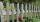Divide the three line segment 13 cm, 26 cm and 19.5 cm long for parts so that the individual parts were equally long and longest. How long will the individual parts and how many it will?
6. Blueberries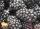Miko and Anton have a total of 1,580 blueberries. Miko and Anton have them in the ratio 2: 3. Determine how much each of them has.
7. 3 masons3 masons received 7,700 CZK. The second half received 1/2 more than the first and third twice more than the second mason. How much they each got crowns?
8. Cows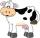Agricultural cooperative has increased the number of housed cows by 14% to 285 units. By how many cows increased agricultural cooperative the number of cows?
9. Jane classWhen asked how many students are in class, Jane said, if we increase the number of students in our class by hundred % and then add half the number of students, we get 100. How many students are in Jane's class?
10. TrapeziumThe lengths of a parallel sides of a trapezium are (2x+3) and (x+8) and the distance between them is (x+4). if the area of the trapezium is 590 , find the value of x.
11. Cube wallsFind the volume and surface area of the cube if the area of one wall is 40cm2.
12. AP - basicsDetermine first member and differentiate of the the following sequence: a3-a5=24 a4-2a5=61
13. Sequence - 5 membersWrite first five members of the sequence ?
14. Z9–I–4 MO 2017Numbers 1, 2, 3, 4, 5, 6, 7, 8 and 9 were prepared for a train journey with three wagons. They wanted to sit out so that three numbers were seated in each carriage and the largest of each of the three was equal to the sum of the remaining two. The conducto
15. Two sisters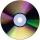Two sisters together have 54 CDs. 6/8 CDs has younger sister and is equal to 3/5 of CDs older sisters. How many CDs has each of the sisters?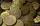Tadeus and Jolana have a total of CZK 15. Jolana has half that of Tadeus. Nevertheless, Jolana has 3 coins and Tadeus 2. Which coin does Tadeus have and which Jolan?
17. Acid solutionBy adding 250 grams of a 96% solution of sulfuric acid to its 3% solution its initial concentration was changed to 25%. How many grams of 3% of the acid were used for dilution?
18. Unknown number 24I think the number: a - is the same as the square area that has the 12th circumfence. What is this number? b - its half is 7 times bigger than its quarter. Is this the number?
19. WineA bottle of wine costs 21 euros; and wine is 20 times more expensive than a bottle. How much a bottle cost?
20. Boxes200 boxes have been straightened in three rows. The first was 13 more than in the second, and in the second was one fifth more than in the third one. How many boxes are in each row?

Do you have an interesting mathematical problem that you can't solve it? Enter it, and we can try to solve it.

To this e-mail address, we will reply solution; solved examples are also published here. Please enter e-mail correctly and check whether you don't have a full mailbox.